Next: Effect of Dielectric Materials Up: Electromagnetic Waves Previous: Maxwell's Equations

## Electromagnetic Waves

One of the first things that Maxwell did with his four equations, once he had obtained them, was to look for wave-like solutions. Maxwell knew that the wave-like solutions of the equations of gas dynamics correspond to sound waves, and the wave-like solutions of the equations of fluid dynamics correspond to gravity waves in water, so he reasoned that if his equations possessed wave-like solutions then these would correspond to a completely new type of wave, which he called an electromagnetic wave.

Maxwell was primarily interested in electromagnetic waves which can propagate through a vacuum (i.e., a region containing no charges or currents). Now, in a vacuum, Maxwell's equations reduce to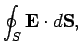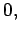(319)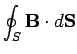(320)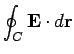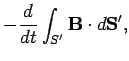(321)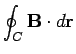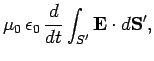(322)

whereis a closed surface, and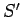a surface attached to some loop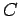. Note that, with the addition of the displacement current term on the right-hand side of Eq. (322), these equations exhibit a nice symmetry between electric and magnetic fields. Unfortunately, Maxwell's mathematical proof that the above equations possess wave-like solutions lies well beyond the scope of this course. We can, nevertheless, still write down these solutions, and comment on them.

Consider a plane electromagnetic wave propagating along the-axis. According to Maxwell's calculations, the electric and magnetic fields associated with such a wave take the form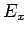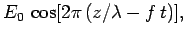(323)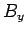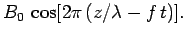(324)

Note that the fields are periodic in both time and space. The oscillation frequency (in hertz) of the fields at a given point in space is. The equation of a wave crest is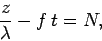(325)

whereis an integer. It can be seen that the distance along the-axis between successive wave crests is given by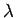. This distance is conventionally termed the wavelength. Note that each wave crest propagates along the-axis. In a time interval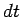, theth wave crest moves a distance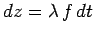, according to Eq. (325). Hence, the velocity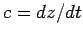with which the wave propagates along the-axis is given by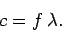(326)

Maxwell was able to establish that electromagnetic waves possess the following properties:

1. The magnetic field oscillates in phase with the electric field. In other words, a wave maximum of the magnetic field always coincides with a wave maximum of the electric field in both time and space.
2. The electric field is always perpendicular to the magnetic field, and both fields are directed at right-angles to the direction of propagation of the wave. In fact, the wave propagates in the direction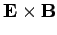. Electromagnetic waves are clearly a type of transverse wave.
3. For a-directed wave, the electric field is free to oscillate in any direction which lies in the-plane. The direction in which the electric field oscillates is conventionally termed the direction of polarization of the wave. Thus, Eqs. (323) represent a plane electromagnetic wave which propagates along the-axis, and is polarized in the-direction.
4. The maximum amplitudes of the electric and the magnetic fields are related via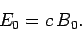(327)

5. There is no constraint on the possible frequency or wavelength of electromagnetic waves. However, the propagation velocity of electromagnetic waves is fixed, and takes the value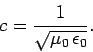(328)

According to Eqs. (321) and (322), a changing magnetic field generates an electric field, and a changing electric field generates a magnetic field. Thus, we can think of the propagation of an electromagnetic field through a vacuum as due to a kind of leap-frog'' effect, in which a changing electric field generates a magnetic field, which, in turn, generates an electric field, and so on. Note that the displacement current term in Eq. (322) plays a crucial role in the propagation of electromagnetic waves. Indeed, without this term, a changing electric field is incapable of generating a magnetic field, and so there can be no leap-frog effect. Electromagnetic waves have many properties in common with other types of wave (e.g., sound waves). However, they are unique in one respect: i.e., they are able to propagate through a vacuum. All other types of waves require some sort of medium through which to propagate.

Maxwell deduced that the speed of propagation of an electromagnetic wave through a vacuum is entirely determined by the constants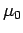and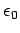[see Eq. (328)]. The former constant is related to the strength of the magnetic field generated by a steady current, whereas the latter constant is related to the strength of the electric field generated by a stationary charge. The values of both constants were well known in Maxwell's day. In modern units,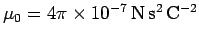and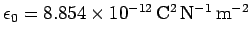. Thus, when Maxwell calculated the velocity of electromagnetic waves he obtained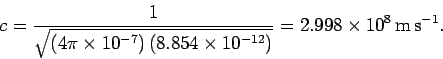(329)

Now, Maxwell knew [from the work of Fizeau (1849) and Foucault (1850)] that the velocity of light was about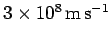. The remarkable agreement between this experimentally determined velocity and his theoretical prediction for the velocity of electromagnetic waves immediately lead Maxwell to hypothesize that light is a form of electromagnetic wave. Of course, this hypothesis turned out to be correct. We can still appreciate that Maxwell's achievement in identifying light as a form of electromagnetic wave was quite remarkable. After all, his equations were derived from the results of bench-top laboratory experiments involving charges, batteries, coils, and currents, etc., which apparently had nothing whatsoever to do with light.

Maxwell was able to make another remarkable prediction. The wavelength of light was well known in the late nineteenth century from studies of diffraction through slits, etc. Visible light actually occupies a surprisingly narrow range of wavelengths. The shortest wavelength blue light which is visible has a wavelength of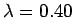microns (one micron is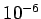meters). The longest wavelength red light which is visible has a wavelength of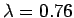microns. However, there is nothing in Maxwell's analysis which suggested that this particular range of wavelengths is special. In principle, electromagnetic waves can have any wavelength. Maxwell concluded that visible light forms a small element of a vast spectrum of previously undiscovered types of electromagnetic radiation.

Since Maxwell's time, virtually all of the non-visible parts of the electromagnetic spectrum have been observed. Table 3 gives a brief guide to the electromagnetic spectrum. Electromagnetic waves are of particular importance because they are our only source of information regarding the Universe around us. Radio waves and microwaves (which are comparatively hard to scatter) have provided much of our knowledge about the centre of the Galaxy. This is completely unobservable in visible light, which is strongly scattered by interstellar gas and dust lying in the galactic plane. For the same reason, the spiral arms of the Galaxy can only be mapped out using radio waves. Infrared radiation is useful for detecting proto-stars which are not yet hot enough to emit visible radiation. Of course, visible radiation is still the mainstay of astronomy. Satellite based ultraviolet observations have yielded invaluable insights into the structure and distribution of distant galaxies. Finally, X-ray and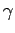-ray astronomy usually concentrates on exotic objects in the Galaxy such as pulsars and supernova remnants.

Table 3: The electromagnetic spectrum.
 Radiation Type Wavelength Range () Gamma Rays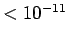X-Rays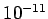-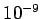Ultraviolet-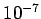Visible-Infrared-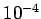Microwave-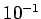TV-FM-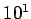Radio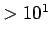Next: Effect of Dielectric Materials Up: Electromagnetic Waves Previous: Maxwell's Equations
Richard Fitzpatrick 2007-07-14